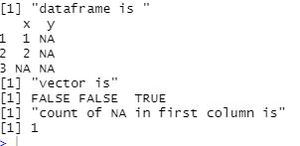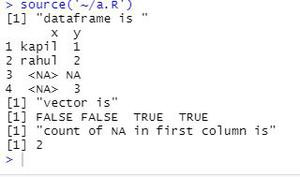Related Articles

# Count the number of NA values in a DataFrame column in R

• Last Updated : 26 Mar, 2021

A null value in R is specified using either NaN or NA. In this article, we will see how can we count these values in a column of a dataframe.

Approach

• Create dataframe
• Pass the column to be checked to is.na() function

Syntax: is.na(column)

Parameter:

column: column to be searched for na values

Returns:

A vector with boolean values, TRUE for NA otherwise FALSE

• From the vector add the values which are TRUE
• Display this number
• Here, 0 means no NA value

Given below are few examples

Example 1:

## R

 `df<-``data.frame``(x = ``c``(1,2,``NA``), y = ``rep``(``NA``, 3))``print``(``"dataframe is "``)``print``(df)`` ` `print``(``"vector is"``)``vec = ``is.na``(df[,1])``print``(vec)`` ` `count = ``sum``(vec)`` ` `print``(``"count of NA in first column is"` `)``print``(count)`

Output:Example 2:

## R

 `df<-``data.frame``(x = ``c``(``"kapil"``,``"rahul"``,``NA``,``NA``), y = ``c``(1,2,``NA``,3))``print``(``"dataframe is "``)``print``(df)`` ` `print``(``"vector is"``)``vec = ``is.na``(df[,1])``print``(vec)`` ` `count = ``sum``(vec)`` ` `print``(``"count of NA in first column is"` `)``print``(count)`

Output:My Personal Notes arrow_drop_up